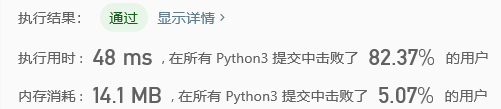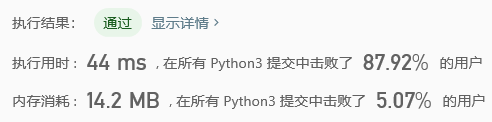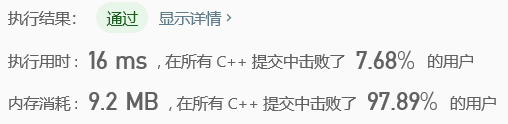# 【LeetCode刷题-简单】167. 两数之和 II - 输入有序数组（python c++）

## 题目描述

•     返回的下标值（index1 和 index2）不是从零开始的。
•     你可以假设每个输入只对应唯一的答案，而且你不可以重复使用相同的元素。

## 思路

### 双指针

• 时间复杂度：O(n)。每个元素最多被访问一次，共有 n 个元素。
• 空间复杂度：O(1)。只是用了两个指针。

## 代码

### python

class Solution:
def twoSum(self, numbers: List[int], target: int) -> List[int]:
left = 1
right= len(numbers)
while(left < right):
left +=1
right-=1
else:
return left, rightclass Solution:
def twoSum(self, numbers: List[int], target: int) -> List[int]:
visited = {}
for index, num in enumerate(numbers):
if target - num not in visited:
visited[num] = index + 1
else:
return visited[target - num], index+1### C++

class Solution {
public:
vector<int> twoSum(vector<int>& numbers, int target) {
int left = 1;
int right= numbers.size();
while(left < right){
int add = numbers[left-1] + numbers[right-1];
if(add == target)   return {left, right};
}
return {-1, -1};
}
};©️2019 CSDN 皮肤主题: 深蓝海洋 设计师: CSDN官方博客